# 用canvas绘制一个曲线动画——深入理解贝塞尔曲线

## 前言

• 它可以用来绘制曲线，在svg和canvas中，原生提供的曲线绘制都是使用贝赛尔曲线
• 它也可以用来描述一个缓动算法，设置css的`transition-timing-function`属性，可以使用贝塞尔曲线来描述过渡的缓动计算
• 几乎所有前端2D或3D图形图表库(echarts，d3，three.js)都会使用到贝塞尔曲线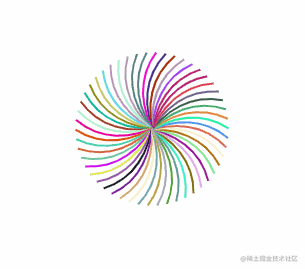实现这样一个曲线动画

## 贝塞尔曲线

### 二次贝塞尔曲线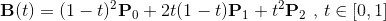• 选定一个`0-1``t`
• 通过`P0``P1`计算出点`Q0``Q0``P0` `P1`连成的直线上，并且`length( P0, Q0 ) = length( P0, P1 ) * t`
• 同样，通过`P1``P2`计算出`Q1`，使得`length( P1, Q1 ) = length( P1, P2 ) * t`
• 再重复一次这个步骤，通过`Q1``Q2`计算出`B`，使得`length( Q0, Q1 ) = length( Q0, B ) * t``B`就为当前曲线上的点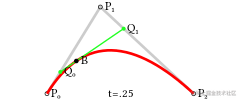图：二次贝塞尔曲线结构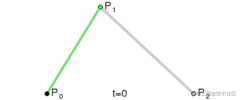图：二次贝塞尔线绘制过程

``ctx.quadraticCurveTo( p1x, p1y, p2x, p2y )复制代码``

### 三次贝塞尔曲线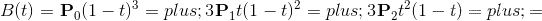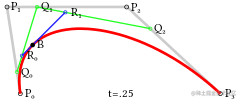图：三次贝塞尔曲线结构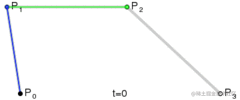图：三次贝塞尔曲线绘制过程

``ctx.bezierCurveTo( p1x, p1y, p2x, p2y, p3x, p3y )复制代码``

### 贝塞尔曲线的特征

#### 四次贝塞尔曲线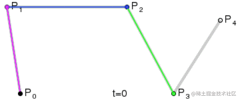#### 五次贝塞尔曲线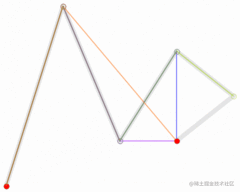图：五次贝塞尔曲线

1. n阶贝塞尔曲线需要n+1个点来确定
2. 贝塞尔曲线是平滑的
3. 贝塞尔曲线的起点和终点与对应控制点的连线相切

## 绘制贝塞尔曲线

``````/**
* 绘制二次贝赛尔曲线路径
* @param  {Object} ctx
* @param  {Array<number>} p0
* @param  {Array<number>} p1
* @param  {Array<number>} p2
*/
function drawCurvePath( ctx, p0, p1, p2 ) {
// ...
}复制代码``````

``````/**
* 绘制二次贝赛尔曲线路径
* @param  {CanvasRenderingContext2D} ctx
* @param  {Array<number>} p0
* @param  {Array<number>} p1
* @param  {Array<number>} p2
*/
function drawCurvePath( ctx, p0, p1, p2 ) {
ctx.moveTo( p0[ 0 ], p0[ 1 ] );
p1[ 0 ], p1[ 1 ],
p2[ 0 ], p2[ 1 ]
);
}复制代码``````

``````/**
* 绘制一条曲线路径
* @param  {CanvasRenderingContext2D} ctx
* @param  {Array<number>} start 起点
* @param  {Array<number>} end 终点
* @param  {number} curveness 曲度(0-1)
*/
function drawCurvePath( ctx, start, end, curveness ) {
// ...
}复制代码``````

``````/**
* 绘制一条曲线路径
* @param  {Object} ctx canvas渲染上下文
* @param  {Array<number>} start 起点
* @param  {Array<number>} end 终点
* @param  {number} curveness 曲度(0-1)
*/
function drawCurvePath( ctx, start, end, curveness ) {
// 计算中间控制点
var cp = [
( start[ 0 ] + end[ 0 ] ) / 2 - ( start[ 1 ] - end[ 1 ] ) * curveness,
( start[ 1 ] + end[ 1 ] ) / 2 - ( end[ 0 ] - start[ 0 ] ) * curveness
];
ctx.moveTo( start[ 0 ], start[ 1 ] );
cp[ 0 ], cp[ 1 ],
end[ 0 ], end[ 1 ]
);
}复制代码``````

``````<!DOCTYPE html>
<html lang="en">
<title>draw curve</title>
<body>
<canvas id="canvas" width="800" height="800"></canvas>
<script>
var canvas = document.getElementById( 'canvas' );
var ctx = canvas.getContext( '2d' );

ctx.lineWidth = 2;
ctx.strokeStyle = '#000';
ctx.beginPath();

drawCurvePath(
ctx,
[ 100, 100 ],
[ 200, 300 ],
0.4
);

ctx.stroke();

function drawCurvePath( ctx, start, end, curveness ) {
// ...
}
</script>
</body>
</html>复制代码``````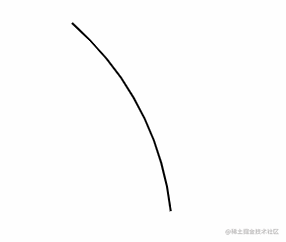## 绘制贝塞尔曲线动画

``````/**
* 绘制一条曲线路径
* @param  {Object} ctx canvas渲染上下文
* @param  {Array<number>} start 起点
* @param  {Array<number>} end 终点
* @param  {number} curveness 曲度(0-1)
* @param  {number} percent 绘制百分比(0-100)
*/
function drawCurvePath( ctx, start, end, curveness, percent ) {
// ...
}复制代码``````### 方法一

``````/**
* 绘制一条曲线路径
* @param  {Object} ctx canvas渲染上下文
* @param  {Array<number>} start 起点
* @param  {Array<number>} end 终点
* @param  {number} curveness 曲度(0-1)
* @param  {number} percent 绘制百分比(0-100)
*/
function drawCurvePath( ctx, start, end, curveness, percent ) {

var cp = [
( start[ 0 ] + end[ 0 ] ) / 2 - ( start[ 1 ] - end[ 1 ] ) * curveness,
( start[ 1 ] + end[ 1 ] ) / 2 - ( end[ 0 ] - start[ 0 ] ) * curveness
];

ctx.moveTo( start[ 0 ], start[ 1 ] );

for ( var t = 0; t <= percent / 100; t += 0.01 ) {

var x = quadraticBezier( start[ 0 ], cp[ 0 ], end[ 0 ], t );
var y = quadraticBezier( start[ 1 ], cp[ 1 ], end[ 1 ], t );

ctx.lineTo( x, y );
}

}

function quadraticBezier( p0, p1, p2, t ) {
var k = 1 - t;
return k * k * p0 + 2 * ( 1 - t ) * t * p1 + t * t * p2;    // 这个方程就是二次贝赛尔曲线方程
}复制代码``````

``````<!DOCTYPE html>
<html lang="en">
<title>draw curve</title>
<body>
<canvas id="canvas" width="800" height="800"></canvas>
<script>
var canvas = document.getElementById( 'canvas' );
var ctx = canvas.getContext( '2d' );

ctx.lineWidth = 2;
ctx.strokeStyle = '#000';

var percent = 0;

function animate() {

ctx.clearRect( 0, 0, 800, 800 );
ctx.beginPath();

drawCurvePath(
ctx,
[ 100, 100 ],
[ 200, 300 ],
0.2,
percent
);

ctx.stroke();

percent = ( percent + 1 ) % 100;

requestAnimationFrame( animate );

}

animate();

function drawCurvePath( ctx, start, end, curveness, percent ) {
// ...
}
</script>
</body>
</html>复制代码``````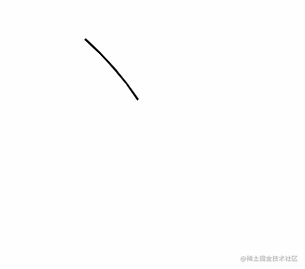### 方法二``````/**
* 绘制一条曲线路径
* @param  {Object} ctx canvas渲染上下文
* @param  {Array<number>} start 起点
* @param  {Array<number>} end 终点
* @param  {number} curveness 曲度(0-1)
* @param  {number} percent 绘制百分比(0-100)
*/
function drawCurvePath( ctx, start, end, curveness, percent ) {

var cp = [
( start[ 0 ] + end[ 0 ] ) / 2 - ( start[ 1 ] - end[ 1 ] ) * curveness,
( start[ 1 ] + end[ 1 ] ) / 2 - ( end[ 0 ] - start[ 0 ] ) * curveness
];

var t = percent / 100;

var p0 = start;
var p1 = cp;
var p2 = end;

var v01 = [ p1[ 0 ] - p0[ 0 ], p1[ 1 ] - p0[ 1 ] ];     // 向量<p0, p1>
var v12 = [ p2[ 0 ] - p1[ 0 ], p2[ 1 ] - p1[ 1 ] ];     // 向量<p1, p2>

var q0 = [ p0[ 0 ] + v01[ 0 ] * t, p0[ 1 ] + v01[ 1 ] * t ];
var q1 = [ p1[ 0 ] + v12[ 0 ] * t, p1[ 1 ] + v12[ 1 ] * t ];

var v = [ q1[ 0 ] - q0[ 0 ], q1[ 1 ] - q0[ 1 ] ];       // 向量<q0, q1>

var b = [ q0[ 0 ] + v[ 0 ] * t, q0[ 1 ] + v[ 1 ] * t ];

ctx.moveTo( p0[ 0 ], p0[ 1 ] );

q0[ 0 ], q0[ 1 ],
b[ 0 ], b[ 1 ]
);

}复制代码``````### 绘制动画